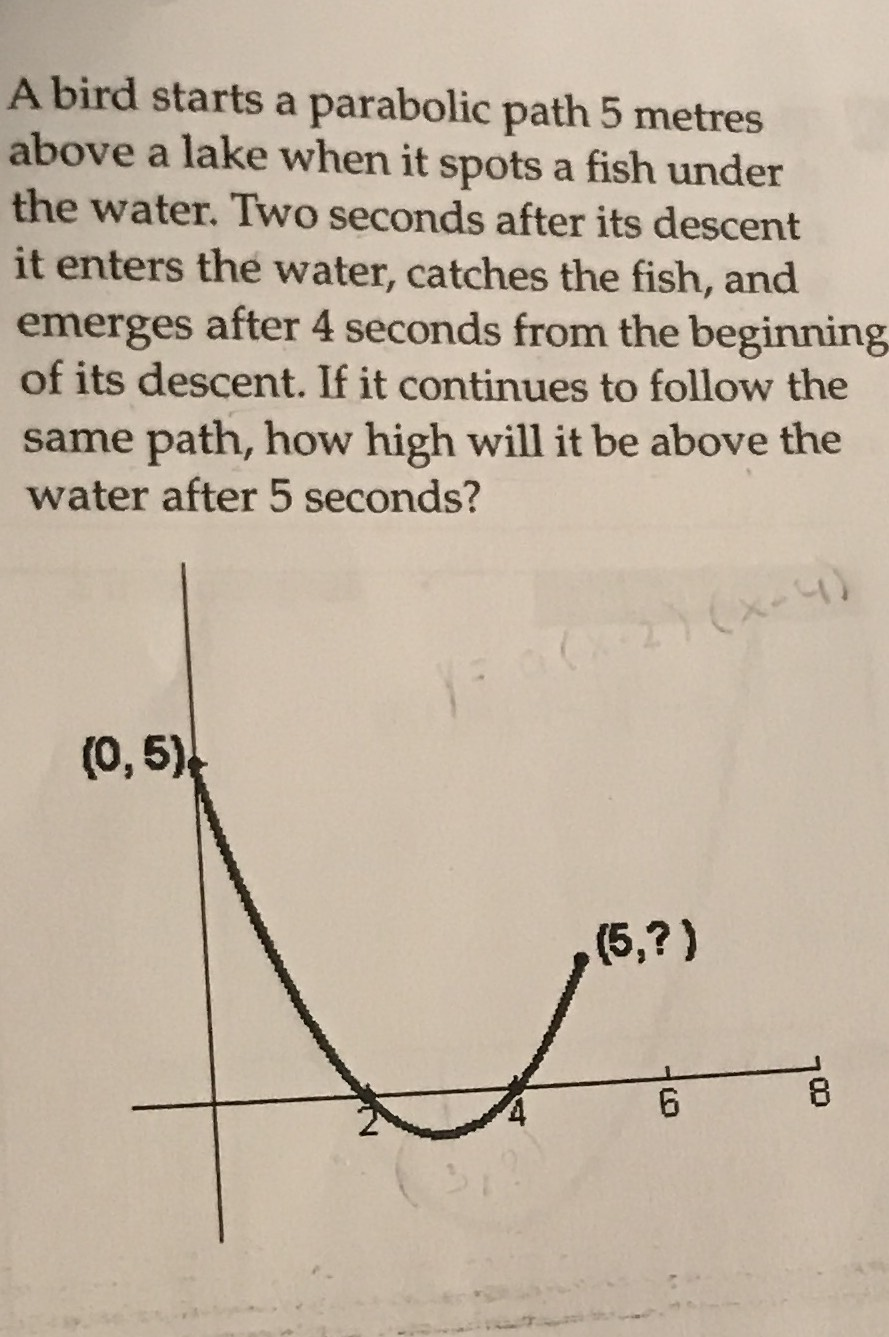### ¿Todavía tienes preguntas de matemáticas?

Pregunte a nuestros tutores expertos
Algebra
PreguntaA bird starts a parabolic path $$5$$ metres above a lake when it spots a fish under the water. Two seconds after its descent it enters the water, catches the fish, and emerges after $$4$$ seconds from the beginning of its descent. If it continues to follow the same path, how high will it be above the water after $$5$$ seconds?

$$y= \frac{5}{8} (x- 3)^2- \frac{5}{8}$$
When x=5, y=$$\frac{15}{8}$$